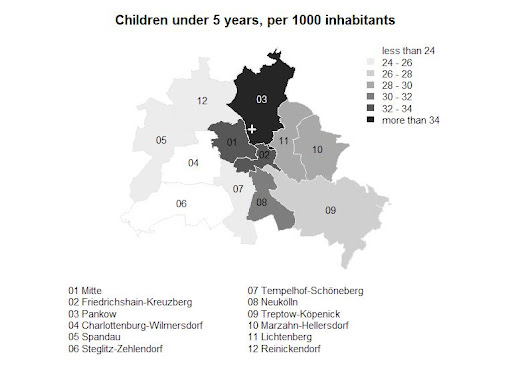## 2012-02-04

### Berlin's children

Few years ago, a newspaper claimed the block I live in — Prenzlauer Berg in Berlin — is the most fertile region in Europe. It was a hoax, as this (German) newspaper article points out. (The article has become quite famous because it coined the term Bionade Biedermeier to describe the life style in this area.)

However, there are more children in my district than in the other parts of Berlin. Have a look at this map:(The base map and population data come from the State’s statistical office. Data at block level is not readily available, though.)

The place I live is marked by a hair cross. Indeed, in this district there is a “higher exposure to kids” than in the other districts, one children per 1000 inhabitants more than in Friedrichshain-Kreuzberg, and twelve children per 1000 more than in Charlottenburg-Wilmersdorf. Exposure is of course different from fertility, maybe that is what I learned from playing with the map.

If you want to know how to draw this thematic map with R, and add the point and the legends to it, or if you are just looking for a shapefile of Berlin, then read on.

The packages required for the plot are `sp`, `classInt` and `RColorBrewer`.

``````> library(sp)
> library(classInt)
> library(RColorBrewer)``````

You need to download the base map as zipped ESRI shapefile and the population data as CSV file (actually it is semicolon separated, use `read.csv2` for import).

After downloading, and in the case of the shapefile, unzipping, the data can be loaded into R and merged:

``````> be.birth <- read.csv2(
+       file="be.birth.csv",
+       colClasses=c(
+         rep("character",2),
+         rep("numeric", 24)
+       )
+   )
> map.be <- readOGR(d, "be_bz")
> map.be@data <- merge(
+     map.be@data,
+     be.birth,
+     by.x="name",
+     by.y="bez",
+     all.x=TRUE
+   )
> map.be@data <- transform(
+     map.be,
+     childr=k5.2010*1000/bev2010
+ )``````

The column `k5.2010` contains the number of children under 5 years, the column `bev2010` is the total population. The dataset contains also the births and the number of women between 15 and 45, for each year from 2005 to 2010, so take a look if you are interested in more.

The next step is to cut the metric variable into categories. I use the `classIntervals` function for that, because it offers several cut methods. Here I am satisfied with the `pretty` method.

``````> plot_var <- map.be@data[, "childr"]
> cuts=8
> plot_pal <- brewer.pal(cuts, "Greys")
> plot_intvl <- classIntervals(plot_var, cuts, style="pretty")
> plot_colors <- findColours(plot_intvl, plot_pal)``````

Now I adjust the margins, plot the map and put the cross hair at my home.

``````> op <- par(mar=c(8,0,3,0))
> plot(map.be, col=plot_colors, border=grey(.9))
> points(x=13.414865, y=52.551463, col=grey(.9), lwd=3, pch=3)
> title("Children under 5 years, per 1000 inhabitants")``````

The map becomes more useful if the districts are labeled. The `coordinates` function returns the center of the districts, which I slightly adjust and then pass to the `text` function.

``````> bz_center <- coordinates(map.be) +
+     matrix(c(
+       0,0,
+       0.018, 0.01,
+       -0.015, 0,
+       rep(0,4),
+       -0.015,0,
+       rep(0,4),
+       0.01, 0.01,
+       0,0.005,
+       0, 0.02,
+       0,0), ncol=2, byrow=TRUE
+     )
> text(
+   bz_center,
+   labels=map.be\$part_ags,
+   cex=0.9,
+   col=c(
+     rep("black",6,),
+     "white",
+     rep("black",4)
+   )
+ )``````

Finally, the legends. On StackOverflow, I learned how to reset the user coordinates and how to allow plotting outside the plot region. Constructing the labels is a bit tricky, and the positions of the legends was found by trial and error.

``````> par(usr=c(0,1,0,1), xpd=NA)
> plot_lbls <- format(
+     round(
+       plot_intvl\$brks[seq(2, length(plot_intvl\$brks)-1)],
+       digits=1),
+     big.mark=".",
+     scientific=FALSE
+ )
> plot_lbls <- c(
+     paste("less than", head(plot_lbls,1)),
+     paste(head(plot_lbls,-1), "-", tail(plot_lbls,-1)),
+     paste("more than", tail(plot_lbls,1))
+ )
> legend(
+   0.7, 1,
+   legend=plot_lbls,
+   fill=attr(plot_colors, "palette"),
+   bty="n",
+   border=attr(plot_colors, "palette"),
+   cex=0.9
+ )
> bz_number <- paste(ifelse(nchar(map.be\$ags)==1, "0", ""),map.be\$ags, sep="")
> bz_lbl <- sort(paste(bz_number, map.be\$name))
> legend(
+  0.1, 0,
+  bz_lbl[1:6],
+  bty="n",
+  cex=0.9
+ )
> legend(
+  0.45, 0,
+  bz_lbl[7:12],
+  bty="n",
+  cex=0.9
+ )``````

And that’s it!

This post has been submitted to R bloggers, a RSS aggregator for R news and tutorials. If you are interested in R, check it out!